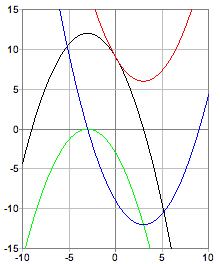First, if you can't remember what the quadratic formula is or you'd like to see a derivation, check out this novel proof.

This is an extensive example, demonstrating an approach to solving multiple quadratic equations, and graphing their functions all at once. Also, a couple of nuances of fnPad semantics are highlighted: floating point results, and dynamic scope.

The first definition is of the quadratic function, where the coefficients are parameters of f(), because we want to graph the function for various different sets of coefficients.

The variable x is "free", meaning that it is defined outside of the application of f() when some particular expressions are "bound" to a, b, and c; implicit definitions. In this example it is bound by graph() for each point of a parabola that is drawn in the window. In general, if a variable v is used in f(), but is not bound by the application of f() (i.e., is not a parameter in the definition), then v is free, and is bound by the application of g(), where the definition of g() uses f(), either directly or indirectly via some intermediate definition, and has v for one of its parameters. When there is no such g(), e.g., when f() is applied directly, then there must be an explicit definition of v or it will be undefined in the application of f(). This is usually called dynamic scoping or binding (as opposed to static scoping, which is more common in programming languages) and is defined formally by the λ notation, the foundation of LISP.

Even if a, b, c, and x are integers the result of calculating f() will be a floating point number since the exponentiation always results in a floating point number, and mixed types thereafter will produce floating points. [Exponentiation of two integers could logically result in an integer, but doesn't happen to, in this version, because of the implementation chosen.]

Then comes the the quadratic formula, broken into a small hierarchy of definitions. First, rootN() and rootP() bind the actual coefficients and use root() to take care of the fact that the quadratic formula has a ± in the middle; rootN() for the root with the negative sign, and of course rootP() for the root with the positive sign. Then root() has most of the formula, depending on only the definition of the discriminant, d. [It wasn't necessary to break down the formula this way, just the habitual technique of good programmers who abhor the duplication of code.]

While a, b, and c are free in root() and d, they are parameters of disc() so that it can be applied directly.

```//========================================================
// In the following, the reason a function has
// a floating point result (if so) is noted.
// ("f.p." is short for floating point.)

//--------------------------------------------------------
// the quadratic formula finds the "roots" of
// (i.e., solves) a*x^2+b*x+c = 0

f(a,b,c) = a*x^2+b*x+c  // x^2 is f.p.; x is "free"

//--------------------------------------------------------

rootN(a,b,c) = root(-1)  // for negative sign
rootP(a,b,c) = root( 1)  // for positive sign
root(sign) = (-b+sign*sqrt(d))/(2*a)  // sqrt(d) is f.p.

// discriminant: real roots when d >= 0

d = b^2-4*a*c  // b^2 is f.p.; a, b, c are "free"
disc(a,b,c) = d

//========================================================
//   a) find the discriminant
//   b) find the roots (if they're real)
//   c) graph the function

//--------------------------------------------------------
// problem #1 (black)

a1 = -1./3; b1 = -2; c1 = 9

disc(a1,b1,c1) ≈ 16.0
rootN(a1,b1,c1) ≈ 3.0; rootP(a1,b1,c1) ≈ -9.0
graph(x,f(a1,b1,c1))

//--------------------------------------------------------
// problem #2 (red) - flip a

a2 = -a1; b2 = b1; c2 = c1

disc(a2,b2,c2) ≈ -8.0
rootN(a2,b2,c2) ≈ NaN; rootP(a2,b2,c2) ≈ NaN
graph(x,f(a2,b2,c2))

//--------------------------------------------------------
// problem #3 (green) - force the discriminant to be 0

a3 = a1; b3 = b1; c3 = -b3^2/(4*a3)

disc(a3,b3,c3) ≈ 0.0
rootN(a3,b3,c3) ≈ -3.0; rootP(a3,b3,c3) ≈ -3.0
graph(x,f(a3,b3,c3))

//--------------------------------------------------------
// problem #4 (blue) - flip a and c

a4 = -a1; b4 = b1; c4 = -c1

disc(a4,b4,c4) ≈ 16.0
rootN(a4,b4,c4) ≈ -3.0; rootP(a4,b4,c4) ≈ 9.0
graph(x,f(a4,b4,c4))

//========================================================
// define the graphs' bounds

graph.x.min = -graph.x.max; graph.x.max = 10
graph.y.min = -graph.y.max; graph.y.max = 15
```Next there is a set of four problems and their solutions with a graph for each. For each problem, some "a", "b", and "c", unique to that problem, are defined: a1, b1, c1 for problem #1; a2, b2, c2 for problem #2; and so on. Then, the discriminant, "positive root", and "negative root" functions are applied to the coefficients.

Note that when there are no real roots (the discriminant < 0) the root functions result in "NaN", which denotes "Not a (real) Number".

Then the graph, shown here, for the quadratic function of each problem is done. The color used for each graph depends on its order in the text. The comments, "(blue)", for example are there as a reminder. In this example, being able to see how each curve is related to the others - offset, reflected - is instructive of the effects of changing particular coefficients.

Last, the bounds of the displayed coordinated system, for all graphs, are defined.